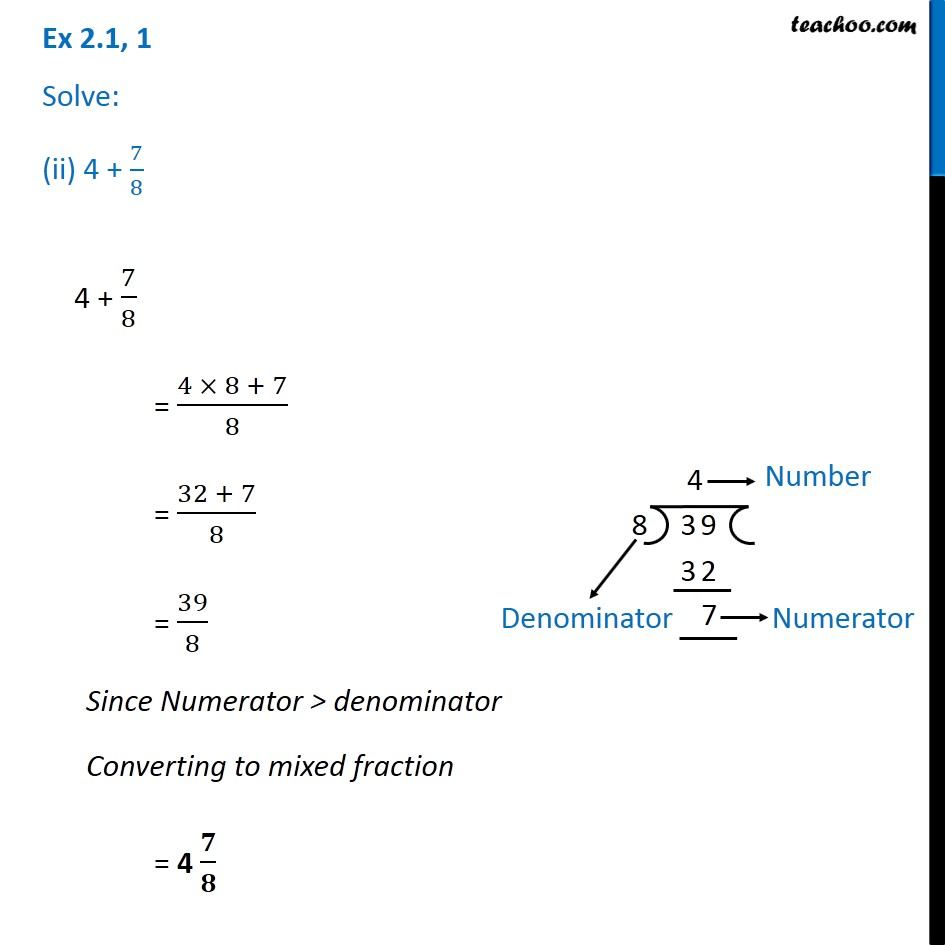Chapter 2 Class 7 Fractions and Decimals
Serial order wiseLearn in your speed, with individual attention - Teachoo Maths 1-on-1 Class

### Transcript

Question 1 Solve: (ii) 4 + 7/8 4 + 7/8 = (4 × 8 + 7)/8 = (32 + 7)/8 = 39/8 Since Numerator > denominator Converting to mixed fraction = 4 𝟕/𝟖